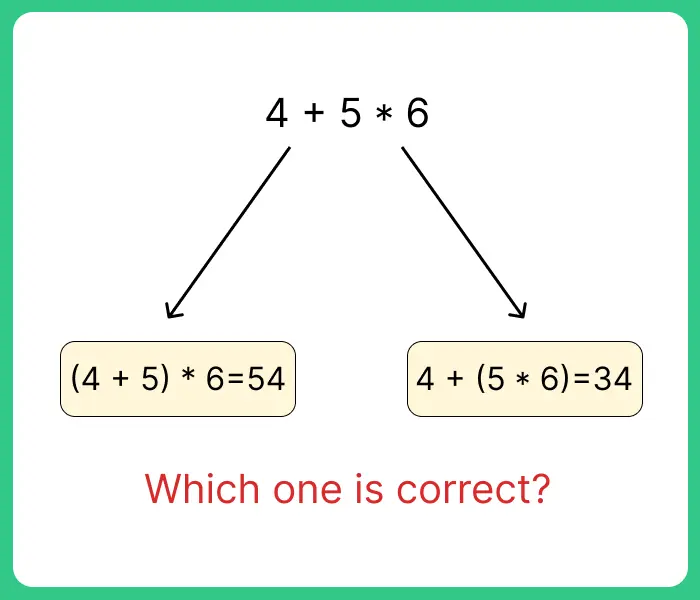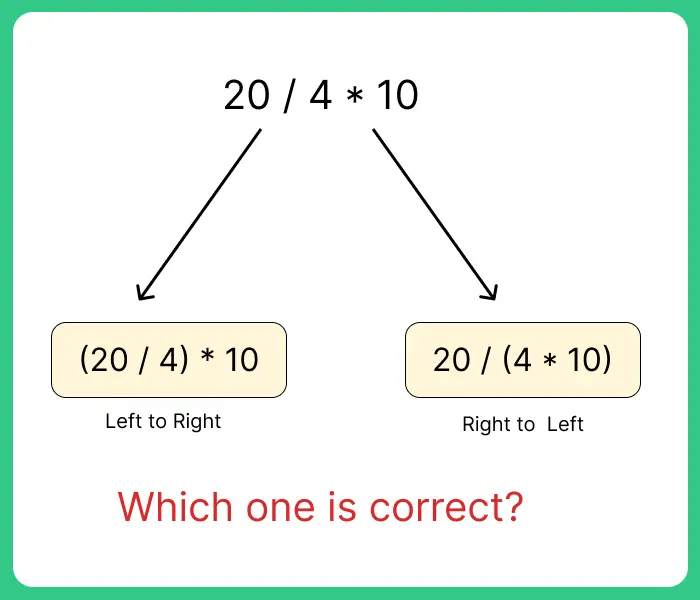# Precedence and Associativity of Operators in C

## Precedence and Associativity:

On this page we will discuss about the Precedence and associativity of operators in C language.Precedence of operator comes into picture when in an expression  we need to decide which  operator will be executed first.Operator with higher precedence will be executed first.If the operators have same precedence in that case associativity comes into play.## Precedence and Associativity of Operators in C Language:

• Precedence of Operators – Suppose, you have multiple operators in an expression.Then which operator will be evaluated first is decided by the precedence.Operator with higher precedence will be evaluated first.
For Example:Therefore, 4+(5*6)= 34 is correct.Whereas,(4+5)*6=54 is wrong.Because precedence of (5*6) is greater so it will be evaluated first then the addition will ne performed.Hence the final answer is 34 and not 54.

• Associativity of Operators – Associativity of operators come into picture when precedence of operators are same and we need to decide which operator will be evaluated first.Associativity can be either left to right(LTR) or right to left(RTL).
For Example:Therefore,(20/4)*10=50 is correct and the 20/(4*10)=1/2 is wrong .Because the associativity of division amd multiplication is from left to right.So, division will be performed first then multiplication.

Parenthesis/brackets( )    [ ]     ->   .   ++    — Left to Right
Unary

! ~  ++   —  +  –  * &  (type) sizeof

Right to Left
Multiplicative*  /  % Left to Right
Bitwise Shift<<   >> Left to Right
Relational<   <=   >    >= Left to Right
Equality==    != Left to Right
Bitwise AND& Left to Right
Bitwise XOR^ Left to Right

#### Lets see an example:

Run

```#include<stdio.h>
int prep ()
{
printf ("Prep");
return 1;
}

int insta ()
{
printf ("Insta");
return 1;
}

int main ()
{
int p;
p = prep () + insta ();
printf ("%d", p);
return 0;
}
```

#### Output:

`PrepInsta2`

Which function is called first? prep() or inst()?

Well according to above output prep( )  is called first but it is not defined whether prep( ) will be called first or whether insta( ) will be called.Behaviour is undefined and output is compiler dependent.

### Related Banners

Get PrepInsta Prime & get Access to all 200+ courses offered by PrepInsta in One Subscription

## Get over 200+ course One Subscription

Courses like AI/ML, Cloud Computing, Ethical Hacking, C, C++, Java, Python, DSA (All Languages), Competitive Coding (All Languages), TCS, Infosys, Wipro, Amazon, DBMS, SQL and others